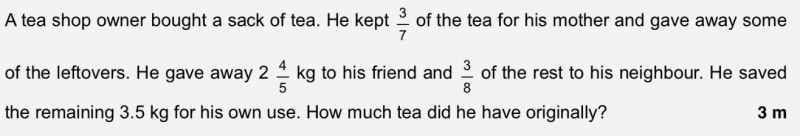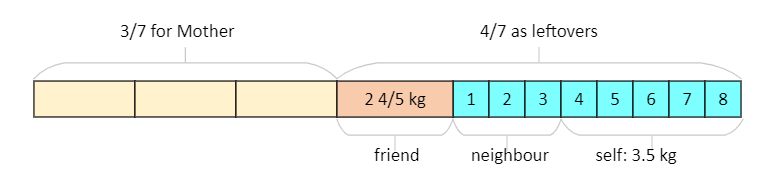# QuestionHelp would be appreciated.

3.5 x8/5 = 5.6
5.6 + 2.8 = 8.4 (this is the 4/7 of original amount)
8.4/4 x 7 = 14.7kg

0 Replies 1 Like```Diagram shows how the tea was distributed. It is easy to work backwards:

5 blue units is 3.5kg
1 blue unit is 3.5/5 = 0.7kg
So leftover = 2 4/5 + 8 x 0.7 = 2.8 + 5.6 = 8.4
Originally he had 8.4 * 7/4 = 14.7kg of tea.

Check:
Mother = 14.7 * 3/7 = 6.3kg
Leftovers = 14.7 - 6.3 = 8.4kg
After giving friend = 8.4 - 2 4/5 = 8.4 - 2.8 = 5.6kg
Neighbour = 3/8 x 5.6 = 2.1kg
Self = 5.6 - 2.1 = 3.5kg```

0 Replies 2 Likes

Total       Kept for mum          Left                  Gave to friend          Rest left

Tea                      7u                 3u                        4u                         2 ⅘  kg                 4u – 2 ⅘

So given to neighbour =  ⅜ ( 4u – 2 ⅘ )

Final left = ⅝ ( 4u – 2 ⅘  )

⅝ ( 4u – 2 ⅘  )  = 3.5  = 3½  =  7/2     ( x 8 on both sides)

5(  4u –  14/5  ) = 28

20u – 14 = 28

u = 42/20 = 2.1

Hence original amount of tea 7u = 7(2.1) = 14.7 kg

Check:

3(2.1) = 6.3

4(2.1) – 2.8 = 5.6

(3/8)(5.6) = 2.1

mom + friend + neighbour + final left = 6.3 + 2.8 + 2.1 + 3.5 = 14.7

0 Replies 1 Like